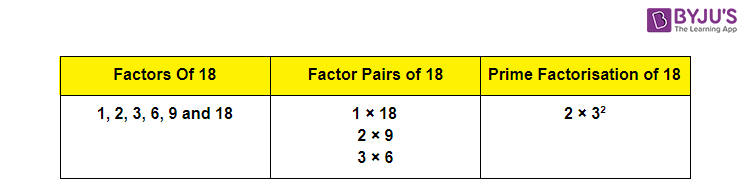# Factors Of 18

Factors of 18 are the numbers that give the original number 18 when multiplied together in pairs. Basically, the factors are the numbers that divide the original number completely. The pair factor of 18 is a pair of numbers on multiplication, resulting in the original number. The pair factor of 18 can be positive as well as negative, but it cannot be a decimal or fraction. For example, the pair factor of 18 is written as (1, 18) or (-1, -18). If we multiply a pair of negative numbers, such as multiplying -1 and -18, results in an original number 18. In this article, we are going to learn what are the factors of 18, pair factors and the prime factors of 18 using the prime factorization method with many solved examples.## What are the Factors of 18?

The factors of 18 are the numbers that divide 18 completely without leaving a remainder value. In other words, the numbers which are multiplied together resulting in the number 18 are the factors of 18. As 18 is an even composite number, it has more factors other than 1 and 18. Hence, the factors of 18 are 1, 2, 3, 6, 9 and 18, and its negative factors are -1, -2, -3, -6, -9 and -18.

 Factors of 18: 1, 2, 3, 6, 9 and 18. Prime Factorization of 18: 2 × 3 × 3 or 2 × 32.

## Pair Factors of 18

The numbers which are multiplied together resulting in 18 is called the pair factor of 18. As discussed above, the pair factors of 18 can be positive or negative. Thus, the positive and negative pair factors of 18 are given below:

Positive Pair Factors of 18:

 Positive Factors of 18 Positive Pair Factors of 18 1 × 18 (1, 18) 2 × 9 (2, 9) 3 × 6 (3, 6)

Negative Pair Factor of 18:

 Negative Factors of 18 Negative Pair Factors of 18 -1 × -18 (-1, -18) -2 × -9 (-2, -9) -3 × -6 (-3, -6)

Hence, the positive pair factors of 18 are (1, 18), (2, 9) and (3, 6). Similarly, the negative pair factors of 18 are (-1, -18), (-2, -9), (-3, -6).

## Factors of 18 by Division Method

In the division method, the factors of 18 are found by dividing 18 by different integers. If the integers divide 18 exactly and leave the remainder zero, then those integers are the factors of 18. Now, let us start dividing 18 by 1 and proceed with the different integers.

• 18/1 = 18 (Factor is 1 and Remainder is 0)
• 18/2 = 9 (Factor is 2 and Remainder is 0)
• 18/3 = 6 (Factor is 3 and Remainder is 0)
• 18/6 = 3 (Factor is 6 and Remainder is 0)
• 18/9 = 2 (Factor is 9 and Remainder is 0)
• 18/18 = 1 (Factor is 18 and Remainder is 0)

If we divide 18 by any numbers other than 1, 2, 3, 6, 9 and 18, it leaves a remainder. Thus, the factors of 18 are 1, 2, 3, 6, 9 and 18.

## Prime Factorization of 18

The number 18 is a composite number. Now let us find the prime factors of it.

• The first step is to divide the number 18 with the smallest prime factor,i.e. 2.

18 ÷ 2 = 9

• Now, check whether 9 can be further divided by 2 or not.

9 ÷ 2 = 4.5

It gives a fractional value. But the factors should be a whole number.

Therefore, we will move to the next prime number, i.e. 3.

• Now, divide 9 by 3.

9 ÷ 3 = 3

• Again divide 3 by 3.

3 ÷ 3 = 1

• We have received 1 at the end and further, we cannot proceed with the division method. So, the prime factors of 18 are 2 × 3 × 3 or we can also write them as 2 × 32, where 2 and 3 both are prime numbers.

## Video Lesson on Prime FactorsLinks Related to Factors Factors And Multiples Factor of 36 Factor Theorem Prime Factors

### Examples

Example 1:

Find the common factors of 18 and 17.

Solution:

The factors of 18 are 1, 2, 3, 6, 9 and 18.

The factors of 17 are 1 and 17.

As 17 is a prime number, the common factor of 18 and 17 is 1.

Example 2:

Find the common factors of 18 and 19.

Solution:

Factors of 18 = 1, 2, 3, 6, 9 and 18.

Factors of 19 = 1 and 19.

The common factor of 18 and 19 is 1, as 19 is a prime number.

Example 3:

Find the common factors of 18 and 9.

Solution:

The factors of 18 are 1, 2, 3, 6, 9 and 18.

The factors of 9 are 1, 3 and 9.

Therefore, the common factors of 18 and 9 are 1, 3 and 9.

Learn the factorization of more numbers here with us in BYJU’S and also download BYJU’S – The Learning App for a better experience.

## Frequently Asked Questions on Factors of 18

Q1

### What are the Factors of 18?

The factors of 18 are 1, 2, 3, 6, 9 and 18.

Q2

### What is the prime factorization of 18?

The prime factorization of 18 is 2 × 3 × 3 or 2 × 32.

Q3

### What are the positive pair factors of 18?

The positive pair factors of 18 are (1, 18), (2, 9) and (3, 6).

Q4

### What are the negative pair factors of 18?

The negative pair factors of 18 are (-1, -18), (-2, -9) and (-3, -6).

Q5

### Is 6 a factor of 18?

Yes, 6 is a factor of 18. As 6 divides 18 completely without leaving a remainder, and hence 6 is a factor of 18.

Quiz on Factors of 18